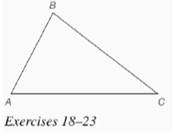Chapter 7.CR, Problem 23CR### Elementary Geometry for College St...

6th Edition
Daniel C. Alexander + 1 other
ISBN: 9781285195698

#### Solutions

Chapter
Section### Elementary Geometry for College St...

6th Edition
Daniel C. Alexander + 1 other
ISBN: 9781285195698
Textbook Problem
1 views

# For Exercises 20 to 23, see the figure for Exercise 18.Use the result from Review Exercise 19 to circumscribe a circle about Δ A B C .To determine

To circumscribe: A circle about triangle ABC.

Explanation

Given:

The triangle ABC is shown below.

Calculation:

Consider the triangle ABC.

The circumcenter X of the triangle ABC is shown below

Having found the circumcenter X, we need the length of the radius to draw a circle...

### Still sussing out bartleby?

Check out a sample textbook solution.

See a sample solution

#### The Solution to Your Study Problems

Bartleby provides explanations to thousands of textbook problems written by our experts, many with advanced degrees!

Get Started

#### Find more solutions based on key concepts